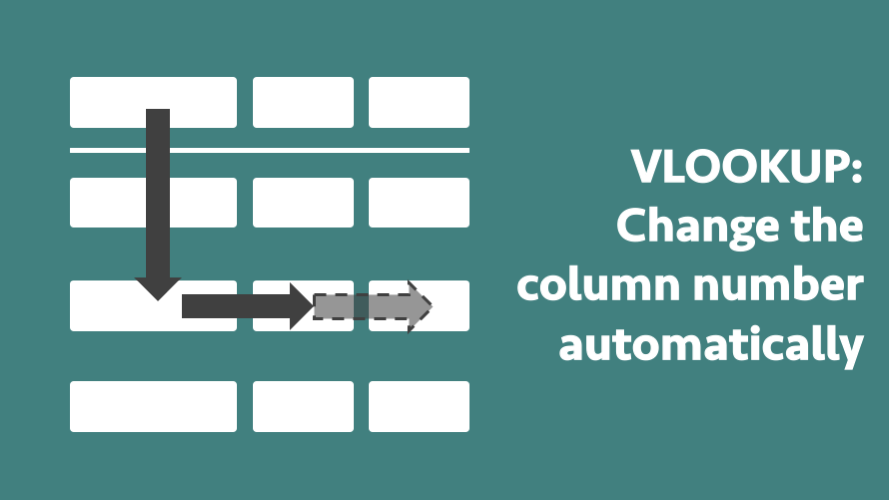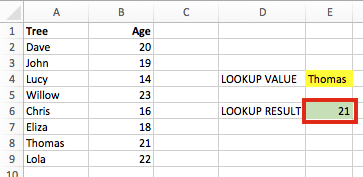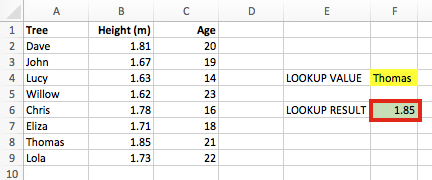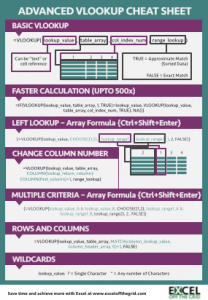Get our FREE VBA eBook of the 30 most useful Excel VBA macros.

Automate Excel so that you can save time and stop doing the jobs a trained monkey could do.

# VLOOKUP: Change the column number automaticallyUnfortunately, VLOOKUP, whilst powerful, simple and easy to use, is one of the least flexible functions in Excel.  Just imagine for a moment that we have entered the following formula into Cell E6 (see screenshot below).

`=VLOOKUP(E4,A2:B10,2,0)`This formula is returning the value from the 2nd column (Column B) – result 21.

But, what happens when a column is inserted between Column A and Column B?  The Range A2:B10 will automatically update to become A2:C10, but the lookup column number will remain the same.  The value will still be returned from the 2nd column, even though that is no longer the column we wish to return the value from.The result has now changed to 1.85, rather than 21.

Assuming we noticed, we now need to go back through each of the VLOOKUP formulas to change the 2 to a 3.  Painful!

We tend to include the column number as a hard-coded number.  But if we can create the column number using formulas then VLOOKUP will be able to update itself when additional columns are inserted.

## Using the COLUMN formula

The COLUMN() formula will return the column number for the cell which it is referring to.  Here are some examples:

`=COLUMN(G2)`

This will return 7, because G is the 7th column

`=COLUMN(Z12)`

This will return 26, because Z is the 26th column

COLUMN only calculates based on the cell reference provided.  By creating a formula using two COLUMN functions we can calculate the column number required.  The format of the formula is:

`=COLUMN([lookup return column])-COLUMN([first column])+1`

Where [first column] and [lookup return column] are the cell references to any cell in that column.  Using our initial example, it would be as follows

`=COLUMN(B1)-COLUMN(A1)+1`

Try it out – this gives us a result of 2.  Now we can replace the column number in our original VLOOKUP function as follows:

`=VLOOKUP(E4,A2:B10,COLUMN(B1)-COLUMN(A1)+1,0)`

If any columns between Column A and Column B are inserted, our entire formula will update itself and still return the correct values.

`=VLOOKUP(E4,A2:C10,COLUMN(C1)-COLUMN(A1)+1,0)`

## Other advantages of using COLUMN in VLOOKUP

This method of using the COLUMN formula has one other big advantage; the formula can now be dragged, copied and moved.

`=VLOOKUP(E\$4,\$A\$2:\$B\$10,COLUMN(B\$1)-COLUMN(\$A\$1)+1,0)`

By inserting the \$ signs into the right places, the column number will change as the formula is copied across.

Download the Advanced VLOOKUP Cheat Sheet.  It includes most of the tips and tricks we’ve covered in this series, including faster calculations, multiple criteria, left lookup and much more.

Please download it and pin it up at work, you can even forward it onto your friends and co-workers.## Other posts in the Mastering VLOOKUP SeriesGet our FREE VBA eBook of the 30 most useful Excel VBA macros.

Automate Excel so that you can save time and stop doing the jobs a trained monkey could do.

Don’t forget:

If you’ve found this post useful, or if you have a better approach, then please leave a comment below.

Do you need help adapting this to your needs?

I’m guessing the examples in this post didn’t exactly meet your situation.  We all use Excel differently, so it’s impossible to write a post that will meet everybody’s needs.  By taking the time to understand the techniques and principles in this post (and elsewhere on this site) you should be able to adapt it to your needs.

But, if you’re still struggling you should:

1. Read other blogs, or watch YouTube videos on the same topic.  You will benefit much more by discovering your own solutions.
2. Ask the ‘Excel Ninja’ in your office.  It’s amazing what things other people know.
3. Ask a question in a forum like Mr Excel, or the Microsoft Answers Community.  Remember, the people on these forums are generally giving their time for free.  So take care to craft your question, make sure it’s clear and concise.  List all the things you’ve tried, and provide screenshots, code segments and example workbooks.
4. Use Excel Rescue, who are my consultancy partner.   They help by providing solutions to smaller Excel problems.

What next?
Don’t go yet, there is plenty more to learn on Excel Off The Grid.  Check out the latest posts:

## 2 thoughts on “VLOOKUP: Change the column number automatically”

1.andrew says:

Thank you for the help. I really appreciate it !!

If helpful to you, I prefer the below method instead of the column function described here.

index(results column, match(lookup value, search column,0))

If you do change your suggested solution, there is no need to thank me or give credit to me.
Cheers,
Andrew

•Excel Off The Grid says:

Hi Andrew – yes, I completely agree. I even wrote an extensive post about it:
https://exceloffthegrid.com/real-reason-index-match-better-vlookup/

Unfortunately, not everybody is a fan of INDEX/MATCHs, VLOOKUP continues. But there is the new XLOOKUP function which may be the best option yet.